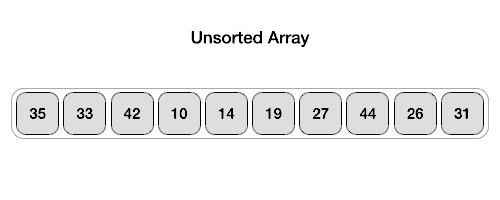## Quick Sort - Data Structures and Algorithms

Quick sort is a highly efficient sorting algorithm and is based on partitioning of array of data into smaller arrays. A large array is partitioned into two arrays one of which holds values smaller than the specified value, say pivot, based on which the partition is made and another array holds values greater than the pivot value.

Quick sort partitions an array and then calls itself recursively twice to sort the two resulting subarrays. This algorithm is quite efficient for large-sized data sets as its average and worst case complexity are of O(n2), where n is the number of items.

## 1. Partition in Quick Sort

Following animated representation explains how to find the pivot value in an array.The pivot value divides the list into two parts. And recursively, we find the pivot for each sub-lists until all lists contains only one element.

## 2. Quick Sort Pivot Algorithm

Based on our understanding of partitioning in quick sort, we will now try to write an algorithm for it, which is as follows.

Step 1 − Choose the highest index value has pivot

Step 2 − Take two variables to point left and right of the list excluding pivot

Step 3 − left points to the low index

Step 4 − right points to the high

Step 5 − while value at left is less than pivot move right

Step 6 − while value at right is greater than pivot move left

Step 7 − if both step 5 and step 6 does not match swap left and right

Step 8 − if left ≥ right, the point where they met is new pivot

## 3. Quick Sort Pivot Pseudocode

The pseudocode for the above algorithm can be derived as :

function partitionFunc(left, right, pivot)

leftPointer = left

rightPointer = right - 1

while True do

while A[++leftPointer] < pivot do

//do-nothing

end while

while rightPointer > 0 && A[--rightPointer] > pivot do

//do-nothing

end while

if leftPointer >= rightPointer

break

else

swap leftPointer,rightPointer

end if

end while

swap leftPointer,right

return leftPointer

end function

## 4. Quick Sort Algorithm

Using pivot algorithm recursively, we end up with smaller possible partitions. Each partition is then processed for quick sort. We define recursive algorithm for quicksort as follows

Step 1 − Make the right-most index value pivot

Step 2 − partition the array using pivot value

Step 3 − quicksort left partition recursively

Step 4 − quicksort right partition recursively

## 6. Quick Sort Pseudocode

To get more into it, let see the pseudocode for quick sort algorithm:

procedure quickSort(left, right)

if right-left <= 0

return

else

pivot = A[right]

partition = partitionFunc(left, right, pivot)

quickSort(left,partition-1)

quickSort(partition+1,right)

end if

end procedure

New
Related